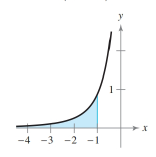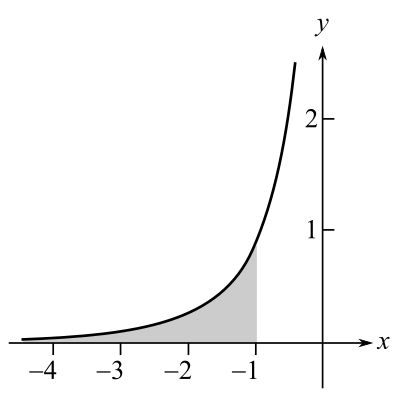Chapter 6.4, Problem 23E### Calculus: An Applied Approach (Min...

10th Edition
Ron Larson
ISBN: 9781305860919

#### Solutions

Chapter
Section### Calculus: An Applied Approach (Min...

10th Edition
Ron Larson
ISBN: 9781305860919
Textbook Problem
1 views

# Area of a Region In Exercises 21-26, find the area of the unbounded shaded region.To determine

To calculate: The area of shaded part for the provide graph:Explanation

Given Information:

The expression is provided as:

7(x1)3dx.

Formula used:

From definition of improper integral.

af(x)dx=limbabf(x)dx

Also, the expression for the integration of a polynomial is as follows:

xndx=xn+1n+1+C

Here, n0.

Calculation:

Consider the provided expression:

7(x1)3dx

For deciding the limit of integral, consider the provided graph,

From the provided graph, lower limit is and upper limit is 1 for the integration.

Use the property of improper integral af(x)dx=limbabf(x)dx and simplify as:

17(x1)3dx=lima(a17(x1)3dx)

Integrate the integrand by substation method as:

Assume x1=u

Differentiate as:

dx=du

Now, substitute the values and integrate as:

7(x1)3dx=7u3du=74u2+C

Again, substitute the value of u

### Still sussing out bartleby?

Check out a sample textbook solution.

See a sample solution

#### The Solution to Your Study Problems

Bartleby provides explanations to thousands of textbook problems written by our experts, many with advanced degrees!

Get Started

#### Find f. f(x) = x2, x 0, f(1) = 0, f(2) = 0

Single Variable Calculus: Early Transcendentals, Volume I

#### In Exercises 49-62, find the indicated limit, if it exists. 51. limx0x2xx

Applied Calculus for the Managerial, Life, and Social Sciences: A Brief Approach

#### In Problems 1-6 simplify each fraction. 1.

Mathematical Applications for the Management, Life, and Social Sciences

#### Simplify: 1240

Elementary Technical Mathematics

#### True or False: f(x) = 10x − x2 is increasing on (4,8).

Study Guide for Stewart's Single Variable Calculus: Early Transcendentals, 8th

#### Simplify. (a2+b2)+(a2+b2)

Mathematics For Machine Technology